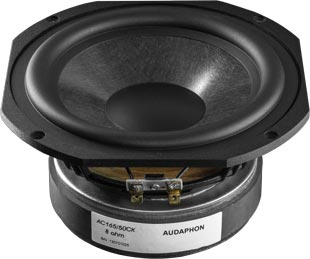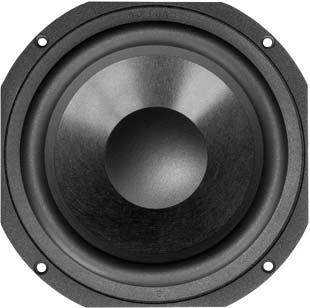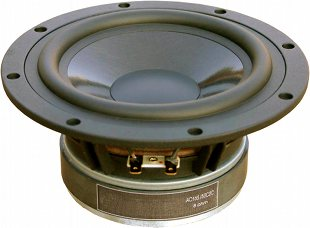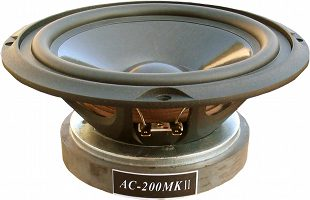# Audaphon

Audaphon is a German brand that searches worldwide for loudspeaker drivers with the highest quality characteristics.

Audaphon drivers feature an excellent price / performance ratio.

Only high-grade materials are used to ensure solidity and best possible sound.

For the high frequency range Audaphon offers

• high quality domes,
• ribbon tweeter
• and Air Motion Transformer
of which the latter can hardly be surpassed in terms of airiness and transparency.

## Wooferrecommended cabinet 1:
closed cabinet with 7,7 L volume
from 78/49 Hz (-3dB/-8dB)

recommended cabinet 2:
31 L volume bass reflex cabinet
with HP70 reflex tube, 16 cm long.
from 37/31 Hz (-3dB/-8dB).

## Audaphon MB 18-8

order no. str-mb18-8

EUR 47,76excl. VAT: € 40.13 / \$ 48.17

16 cm bass driver with paper cone. To avoid compression effects the driver possesses a pole piece hole. The basket is made of die-cast.

• power handling (continuous/programme) = 70/140 W
• resonance frequency fs = 44,5 Hz
• impedance R = 8 Ohm
• sound pressure level SPL = 87 dB (2,83V; 1m)
• DC resistance Re = 7,3 Ohm
• force factor BL = 8,11 N/A
• voice coil inductance L = 0,75 mH
• effective piston radiating area Sd = 133 cm2
• effective mechanical mass incl. air load mms = 16 g
• equivalent volume of compliance Vas = 19 l
• total Q factor Qts = 0,46 (Qms=6,5, Qes=0,49)
• voice coil diameter = 30 mm
• maximum peak linear excursion vibration xlin = +/- 2,5 mm
• mounting diameter d = 145 mm
• overall diameter d = 180 mm
• mounting depth (not countersunk) t = 83 mm
• mass m = 2,2 kgopen a bigger photo

recommended cabinet 1:
closed cabinet with 17 L volume
from 69/44 Hz (-3dB/-8dB)

recommended cabinet 2:
61 L volume bass reflex cabinet
with HP70 reflex tube, 9 cm long.
from 35/28 Hz (-3dB/-8dB).

## Audaphon AC 165

order no. au-ac165

EUR 96,50excl. VAT: € 81.09 / \$ 97.33

• power handling (continuous/programme) = 60/120 W
• resonance frequency fs = 32 Hz
• impedance R = 8 Ohm
• sound pressure level SPL = 89 dB (2,83V; 1m)
• DC resistance Re = 7 Ohm
• force factor BL = 5,3 N/A
• voice coil inductance L = 0,35 mH
• effective piston radiating area Sd = 133 cm2
• effective mechanical mass incl. air load mms = 9,6 g
• equivalent volume of compliance Vas = 76 l
• total Q factor Qts = 0,37 (Qms=3,38, Qes=0,41)
• maximum peak linear excursion vibration xlin = +/- 5 mm
• mounting diameter d = 148 mm
• overall diameter d = 178 (163x163) mm
• mounting depth (not countersunk) t = 75,5 mm
• mass m = 1,35 kgopen a bigger photoopen a bigger photo

recommended cabinet 1:
closed cabinet with 1,3 L volume
from 152/96 Hz (-3dB/-8dB)

recommended cabinet 2:
2,3 L volume bass reflex cabinet
with HP50 reflex tube, 38 cm long.
from 103/76 Hz (-3dB/-8dB).

## Audaphon AC 165/50CK (square basket)

order no. au-ac16550ck-square

EUR 125,75excl. VAT: € 105.67 / \$ 126.83

• power handling (continuous/programme) = 150/300 W
• resonance frequency fs = 37 Hz
• impedance R = 8 Ohm
• sound pressure level SPL = 89 dB (2,83V; 1m)
• DC resistance Re = 7 Ohm
• force factor BL = 12,3 N/A
• voice coil inductance L = 0,67 mH
• effective piston radiating area Sd = 133 cm2
• effective mechanical mass incl. air load mms = 13,7 g
• equivalent volume of compliance Vas = 24 l
• total Q factor Qts = 0,19 (Qms=3,68, Qes=0,2)
• maximum peak linear excursion vibration xlin = +/- 6 mm
• mounting diameter d = 148 mm
• overall diameter d = 178 (163x163) mm
• mounting depth (not countersunk) t = 82,5 mm
• mass m = 2,4 kgopen a bigger photo

recommended cabinet 1:
closed cabinet with 1,7 L volume
from 152/96 Hz (-3dB/-8dB)

recommended cabinet 2:
3 L volume bass reflex cabinet
with HP50 reflex tube, 28 cm long.
from 103/76 Hz (-3dB/-8dB).

## Audaphon AC 165/50C2C

order no. au-ac16550c2c

EUR 135,50excl. VAT: € 113.87 / \$ 136.67

• power handling (continuous/programme) = 150/300 W
• resonance frequency fs = 37 Hz
• impedance R = 8 Ohm
• sound pressure level SPL = 90 dB (2,83V; 1m)
• DC resistance Re = 7 Ohm
• force factor BL = 10,8 N/A
• voice coil inductance L = 0,67 mH
• effective piston radiating area Sd = 133 cm2
• effective mechanical mass incl. air load mms = 14,3 g
• equivalent volume of compliance Vas = 32 l
• total Q factor Qts = 0,19 (Qms=4,22, Qes=0,2)
• maximum peak linear excursion vibration xlin = +/- 6 mm
• mounting diameter d = 151 mm
• overall diameter d = 185 mm
• mounting depth (not countersunk) t = 82,5 mm
• mass m = 2,6 kgopen a bigger photo

recommended cabinet 1:
closed cabinet with 6,2 L volume
from 93/59 Hz (-3dB/-8dB)

recommended cabinet 2:
18 L volume bass reflex cabinet
with HP70 reflex tube, 21 cm long.
from 51/39 Hz (-3dB/-8dB).

## Audaphon AC 180/50C2C

order no. au-ac18050c2c

EUR 154,99excl. VAT: € 130.24 / \$ 156.33

• power handling (continuous/programme) = 150/300 W
• resonance frequency fs = 35 Hz
• impedance R = 8 Ohm
• sound pressure level SPL = 90 dB (2,83V; 1m)
• DC resistance Re = 7 Ohm
• force factor BL = 9 N/A
• voice coil inductance L = 0,67 mH
• effective piston radiating area Sd = 168 cm2
• effective mechanical mass incl. air load mms = 19,5 g
• equivalent volume of compliance Vas = 45 l
• total Q factor Qts = 0,3 (Qms=3,57, Qes=0,33)
• maximum peak linear excursion vibration xlin = +/- 6 mm
• mounting diameter d = 165,5 mm
• overall diameter d = 204 (184x184) mm
• mounting depth (not countersunk) t = 87 mm
• mass m = 2,51 kgopen a bigger photo

recommended cabinet 1:
closed cabinet with 8,4 L volume
from 86/54 Hz (-3dB/-8dB)

recommended cabinet 2:
19 L volume bass reflex cabinet
with HP70 reflex tube, 26 cm long.
from 53/40 Hz (-3dB/-8dB).

## Audaphon AC 200MK2

order no. au-ac200mk2

EUR 164,74excl. VAT: € 138.44 / \$ 166.16

• power handling (continuous/programme) = 150/300 W
• resonance frequency fs = 25 Hz
• impedance R = 8 Ohm
• sound pressure level SPL = 90 dB (2,83V; 1m)
• DC resistance Re = 7 Ohm
• force factor BL = 10,6 N/A
• voice coil inductance L = 0,67 mH
• effective piston radiating area Sd = 220 cm2
• effective mechanical mass incl. air load mms = 29,2 g
• equivalent volume of compliance Vas = 108 l
• total Q factor Qts = 0,24 (Qms=3,99, Qes=0,25)
• maximum peak linear excursion vibration xlin = +/- 8 mm
• mounting diameter d = 182 mm
• overall diameter d = 222 mm
• mounting depth (not countersunk) t = 97 mm
• mass m = 3,01 kgopen a bigger photo

recommended cabinet 1:
closed cabinet with 13,1 L volume
from 82/52 Hz (-3dB/-8dB)

recommended cabinet 2:
33 L volume bass reflex cabinet
with HP70 reflex tube, 14 cm long.
from 48/36 Hz (-3dB/-8dB).

## Audaphon AC 200/50C2C

order no. au-ac20050c2c

EUR 174,49excl. VAT: € 146.63 / \$ 175.99

• power handling (continuous/programme) = 150/300 W
• resonance frequency fs = 27 Hz
• impedance R = 8 Ohm
• sound pressure level SPL = 90 dB (2,83V; 1m)
• DC resistance Re = 7 Ohm
• force factor BL = 8,8 N/A
• voice coil inductance L = 0,67 mH
• effective piston radiating area Sd = 220 cm2
• effective mechanical mass incl. air load mms = 20,2 g
• equivalent volume of compliance Vas = 130 l
• total Q factor Qts = 0,26 (Qms=4,25, Qes=0,28)
• maximum peak linear excursion vibration xlin = +/- 6 mm
• mounting diameter d = 190 mm
• overall diameter d = 225 mm
• mounting depth (not countersunk) t = 98 mm
• mass m = 2,57 kgopen a bigger photo

recommended cabinet 1:
closed cabinet with 29 L volume
from 55/35 Hz (-3dB/-8dB)

recommended cabinet 2:
81 L volume bass reflex cabinet
with HP100 reflex tube, 30 cm long.
from 31/24 Hz (-3dB/-8dB).

## Audaphon AC 250F1

order no. au-ac250f1

EUR 145,24excl. VAT: € 122.05 / \$ 146.49

• power handling (continuous/programme) = 150/300 W
• resonance frequency fs = 20 Hz
• impedance R = 8 Ohm
• sound pressure level SPL = 89 dB (2,83V; 1m)
• DC resistance Re = 7 Ohm
• force factor BL = 11,8 N/A
• voice coil inductance L = 0,67 mH
• effective piston radiating area Sd = 346 cm2
• effective mechanical mass incl. air load mms = 64,5 g
• equivalent volume of compliance Vas = 224 l
• total Q factor Qts = 0,29 (Qms=2,75, Qes=0,32)
• maximum peak linear excursion vibration xlin = +/- 8 mm
• mounting diameter d = 232 mm
• overall diameter d = 278 mm
• mounting depth (not countersunk) t = 116 mm
• mass m = 3,94 kg

The cabinet volume calculations are executed considering an external resistance of 0.3 Ohm. We are using, if no other data is known or available, the information supplied by the manufacturer.

Please note: the frequency response doesn't say much about the cut-off frequency inside the cabinet! Therefore, we mostly give you 2 examples with dimensioning considerations and an indication, how low the loudspeaker will reproduce.

Home